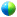19th Symposium on Boundary Layers and Turbulence

11B.6

A local model of the subfilter-scale energy for LES of the atmospheric boundary layer

Scott T. Salesky, The Pennsylvania State University, University Park, PA; and M. Chamecki

Knowledge of the subfilter-scale (SFS) energy is valuable for modeling purposes in large-eddy simulations (LES) of the atmospheric boundary layer (ABL) and for the post processing of LES data. The SFS energy must be known if one wishes to recover the true pressure field from the modified pressure used in simulations, and may be used to form an eddy viscosity in models of the SFS stress. In addition, a model of the SFS energy is also necessary to close the equation set in LES when compressibility effects cannot be neglected. (This is the case in most mesoscale and general circulation models, which use an anelastic approximation or are fully compressible).

The traditional method of determining the SFS energy is through a one-equation model that solves its rate equation numerically. While this approach is common, it requires closure assumptions and has a relatively high computational expense. A number of algebraic models for the SFS energy have been proposed, including dimensional models and models based on the integration of the energy spectrum. These models are global in nature, however, and therefore are only able to predict the average SFS energy. A local model for the SFS energy is proposed for LES of the ABL. The SFS energy model is based on scale similarity and is derived from a stability-dependent model of the energy spectrum in the atmosphere that accounts for buoyancy and shear and therefore is able to account for the shape of the production range of the spectrum.

Results from an a priori test using atmospheric data from the Horizontal Array Turbulence Study (HATS) reveal that the model performs well both in a global and a local sense. The model is able to correctly predict the average SFS energy for stabilities of -1.0 ≤ z/L ≤ 0.5 and nondimensional filter widths of Δ/z = 2.0, 1.0, and 0.5. The performance of the proposed model is more accurate than a similar model that assumes an infinite κ-5/3 spectrum and is unable to account for buoyancy and shear. An examination of PDFs and joint PDFs of the true SFS energy and the ones obtained from the atmospheric model reveal that the model also predicts the instantaneous SFS energy correctly under most conditions, except for stable conditions (z/L > 0.5) when large filter widths (Δ/z = 2.0) are used. This procedure is further extended to model the SFS temperature variance, which demonstrates that this type of local model is applicable to the SFS variance of any passive scalar in LES and therefore has implications for atmospheric dispersion modeling.Recorded presentation

Session 11B, Boundary-layer Processes V
Friday, 6 August 2010, 10:30 AM-12:00 PM, Torrey's Peak III & IV

Previous paper

Browse or search entire meeting